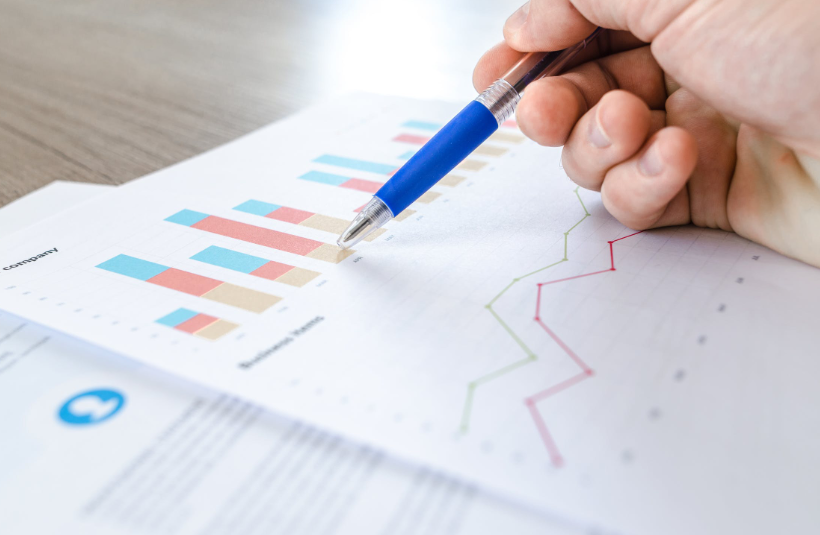# Uses of standard deviation in real lifeAs students, a majority of us hated math not only because of its sheer complexity (debatable) but because of how impractical and useless it seemed to us. “Where does differentiation even mean?” or “Where is standard deviation even used?” were questions most of us asked. As a third-grader, multiplication seemed like a nuisance, as a high school student standard deviation made no sense either. Most of us just memorized the formulae and somehow got done with high school, leaving all those unrealistic mathematical phenomena behind for good.

Little did we know that math and statistics would pop back up in most of our professional careers, from accounting and finance to medical research. Don’t believe it? Read on to find out the numerous practical applications of a simple statistical principle to prove yourself wrong: standard deviation

A quick recap for you: Standard deviation is the measure of dispersion around an average. In simple terms, it shows the spread of data around the average in a given sample. The higher the standard deviation, the higher is the deviation from the mean.  Let us not go into its calculation so that no one leaves half-way through this article.

Following are the uses of standard deviation in real life:

1.     In Finance

Standard deviation is an important tool financial analysts and business-owners use for risk-management and decision-making. Potent risk management maneuvers can be devised in situations like slumping sales or spike in bad customer reviews.

In business risk management procedures, financial analysts use standard deviation to calculate the volatility of stock prices and to calculate margins of error in surveys taken by the company.

For example, you have a transportation and logistics business. To calculate the amount of drivers you need, standard deviation is used.

The Sharpe Ratio, one of the most important ratios in portfolio management, uses standard deviation to calculate risk-adjusted return which helps provide incentives to portfolio managers to gain results while taking the minimum risk possible.

In a company, there is a constant tussle between employees that they get paid less than others and claim that it is unfair on the part of the employer. The employer will then check for disparities by calculating the average salary and its standard deviation for employees in that department. If the standard deviation is higher than expected, the matter will be looked into. For example, when going through the accounts, the employer will realize that the data is skewed because three of the employees are almost 10 years senior to the others and get paid more.

1.     Quality Control

Quality control in production and manufacturing is vital to keep up standards. It is done by testing output samples against a standard. If the standard deviation is greater than expected, the products are rejected as they do not meet the standards set by the particular company.

Measurements as simple as the sugar content and amount of soft drink in a coke can is monitored using standard deviation. For example, if a can of coke has a mean amount of 250 ml and ±2ml is the standard deviation, the minimum amount of coke in a can can be 248ml and the maximum can be 252ml. Anything greater or lesser than that cannot be distributed by the company.

1.     Medicine

In medical research and experimental studies, data is collected and a mathematical model like the normal distribution is applied to it to prove a hypothesis. To explain those statistical analysis results, standard deviation is used. Standard deviation in medicine? You wouldn’t have thought about it, right?

For example, a new anti-viral is being tested for a new virus like the coronavirus. A large number of samples of the virus will be tested with the anti-viral and the experiment will be monitored over time. The mean of the virus elimination rate will be calculated along with standard deviation to prove the anti-viral has the same effect on each sample.

1.     Polling

Polls like who will win in the presidential elections are very common. The margin of error in these polls are calculated using standard deviation. If you ask two groups of 100 people each about who they are going to vote for, you can use the two samples to calculate the difference and the margin of error. It will basically tell you how reliable the poll is.

1.   In Daily Life

There are so many instances in our daily lives where we are applying mathematical phenomena like the standard deviation without even knowing. Teachers calculate standard deviation and mean when they take tests. Weather forecasts make use of standard deviation to find out if their forecast is reliable. In everyday budgeting, you can set a mean amount of money for you to spend and check if you’re spending too much using standard deviation. You will obviously not go around doing calculations, it’s simply an instinctual calculation your mind does for you.

As you see, math and statistics are not as useless as you might have thought before. Which application shocked you the most?

Standard deviation calculations are little complex. Use any online standard deviation calculator to make sure that your calculation are correct.